Foxtable(狐表)用户栏目专家坐堂 → [求助]统计分行填充了，怎么处理？

共有709人关注过本帖树形打印复制链接

# 主题：[求助]统计分行填充了，怎么处理？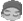cqlpjks
1楼 | 信息 | 搜索 | 邮箱 | 主页 | UC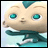加好友发短信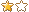[求助]统计分行填充了，怎么处理？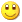Post By：2019/11/11 10:15:00 [只看该作者]

Dim Values() As String = {"总分"}
Dim s1() As String = New String() {"总分"}
Dim dt As Table = Tables("成绩库")
For Each Value As String In Values
Dim nj As String = "2020"
Dim tjlb As String = "4期"
Dim bm As String = Value & "重点人数对照表"
Dim dtb As New DataTableBuilder(bm)
For Each tjbb As String In DataTables("成绩库").GetValues("部别","[年级] = '" & nj & "'And [类别] = '"& tjlb &"'")
For i As Integer = 0 To 2
dtb.AddDef( tjbb & i ,Gettype(Double),"",tjbb &"_"& (nj-i) & "级")
Next
Next
For i As Integer = 0 To 2
dtb.AddDef("合计"& i ,Gettype(Double),"","合  计_"& (nj-i) & "级")
Next
dtb.Build()
For i As Integer = 0 To 2
For Each tjbb As String In DataTables("成绩库").GetValues("部别","[年级] = '" & nj & "'And [类别] = '"& tjlb &"'")
Dim dr As DataRow
dr("单位") = "全区合计"
dr("统计类别") = tjlb
Dim wdr As DataRow = DataTables("分数线").Find("类别 = '" & tjlb & "'And 部别 = '" & tjbb & "'")
Dim tj As String = Left(bm,2) & "排名"
Dim tj1 As String = Left(bm,2)
dr( tjbb & i ) = DataTables("成绩库").Compute("count(单位)", "" & tj1 & " >= '" & wdr("目标" & (i+1) ) & "' And [部别] = '" & tjbb & "' And [类别] = '" & tjlb & "'And [年级] = '"& (nj-i) &"'")
For Each tjdw As String In DataTables("成绩库").GetValues("单位","[年级] = '" & nj & "'And [类别] = '"& tjlb &"'")
dr("单位") = tjdw
dr("统计类别") = tjlb
tj = Left(bm,2) & "排名"
tj1 = Left(bm,2)
dr( tjbb & i ) = DataTables("成绩库").Compute("count(单位)", "" & tj1 & " >= '" & wdr("目标" & (i+1) ) & "' And [单位] = '" & dr("单位") & "' And [部别] = '" & tjlb & "' And [类别] = '" & tjlb & "'And [年级] = '"& (nj-i) &"'")
Next
If bm <> "成绩库" AndAlso bm <> "校名设置" AndAlso bm <> "目标设置" AndAlso bm <> "分数线" AndAlso bm <> "市级1分段" AndAlso bm <> "区县基数" AndAlso bm <> "基数" AndAlso bm <> "调用基数"
dr("单位") = "市级分数线"
dr("统计类别") = tjlb
dr( tjbb & i ) =  wdr("目标" & (i+1) )
End If
Next

Next
Next
StatusBar.Reset
Tables("成绩库").ResumeRedraw

 下载信息  [文件大小：   下载次数： ]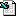点击浏览该文件:统计表结果情况.xls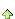2楼 | 信息 | 搜索 | 邮箱 | 主页 | UC加好友发短信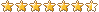Post By：2019/11/11 10:25:00 [只看该作者]

 For Each tjbb As String In DataTables("成绩库").GetValues("部别","[年级] = '" & nj & "'And [类别] = '"& tjlb &"'")            Dim dr As DataRow            dr = DataTables(bm).addnew()            dr("单位") = "全区合计"            dr("统计类别") = tjlb            Dim wdr As DataRow = DataTables("分数线").Find("类别 = '" & tjlb & "'And 部别 = '" & tjbb & "'")            Dim tj As String = Left(bm,2) & "排名"            Dim tj1 As String = Left(bm,2)            dr( tjbb & i ) = DataTables("成绩库").Compute("count(单位)", "" & tj1 & " >= '" & wdr("目标" & (i+1) ) & "' And [部别] = '" & tjbb & "' And [类别] = '" & tjlb & "'And [年级] = '"& (nj-i) &"'")            For Each tjdw As String In DataTables("成绩库").GetValues("单位","[年级] = '" & nj & "'And [类别] = '"& tjlb &"'")dr = DataTables(bm).find("单位='" & tjdw & "'")if dr is nothing then                dr = DataTables(bm).addnew()                dr("单位") = tjdwend if                dr("统计类别") = tjlb                tj = Left(bm,2) & "排名"                tj1 = Left(bm,2)                dr( tjbb & i ) = DataTables("成绩库").Compute("count(单位)", "" & tj1 & " >= '" & wdr("目标" & (i+1) ) & "' And [单位] = '" & dr("单位") & "' And [部别] = '" & tjlb & "' And [类别] = '" & tjlb & "'And [年级] = '"& (nj-i) &"'")            Next            If bm <> "成绩库" AndAlso bm <> "校名设置" AndAlso bm <> "目标设置" AndAlso bm <> "分数线" AndAlso bm <> "市级1分段" AndAlso bm <> "区县基数" AndAlso bm <> "基数" AndAlso bm <> "调用基数"                dr = DataTables(bm).addnew()                dr("单位") = "市级分数线"                dr("统计类别") = tjlb                dr( tjbb & i ) =  wdr("目标" & (i+1) )            End If        Nextcqlpjks
3楼 | 信息 | 搜索 | 邮箱 | 主页 | UC加好友发短信Post By：2019/11/11 11:07:00 [只看该作者]

 For i As Integer = 0 To 2        For Each tjbb As String In DataTables("成绩库").GetValues("部别","[年级] = '" & nj & "'And [类别] = '"& tjlb &"'")            Dim dr As DataRow            dr = DataTables(bm).find("单位='全区合计'")            If dr Is Nothing Then                dr = DataTables(bm).addnew()                dr("单位") = "全区合计"                dr("统计类别") = tjlb            End If            Dim wdr As DataRow = DataTables("分数线").Find("类别 = '" & tjlb & "'And 部别 = '" & tjbb & "'")            Dim tj As String = Left(bm,2) & "排名"            Dim tj1 As String = Left(bm,2)            dr( tjbb & i ) = DataTables("成绩库").Compute("count(单位)", "" & tj1 & " >= '" & wdr("目标" & (i+1) ) & "' And [部别] = '" & tjbb & "' And [类别] = '" & tjlb & "'And [年级] = '"& (nj-i) &"'")            For Each tjdw As String In DataTables("成绩库").GetValues("单位","[年级] = '" & nj & "'And [类别] = '"& tjlb &"'")                dr = DataTables(bm).find("单位='" & tjdw & "'")                If dr Is Nothing Then                    dr = DataTables(bm).addnew()                    dr("单位") = tjdw                End If                dr("统计类别") = tjlb                tj = Left(bm,2) & "排名"                tj1 = Left(bm,2)                dr( tjbb & i ) = DataTables("成绩库").Compute("count(单位)", "" & tj1 & " >= '" & wdr("目标" & (i+1) ) & "' And [单位] = '" & dr("单位") & "' And [部别] = '" & tjlb & "' And [类别] = '" & tjlb & "'And [年级] = '"& (nj-i) &"'")            Next            If bm <> "成绩库" AndAlso bm <> "校名设置" AndAlso bm <> "目标设置" AndAlso bm <> "分数线" AndAlso bm <> "市级1分段" AndAlso bm <> "区县基数" AndAlso bm <> "基数" AndAlso bm <> "调用基数"                dr = DataTables(bm).find("单位= '市级分数线'")                If dr Is Nothing Then                    dr = DataTables(bm).addnew()                    dr("单位") = "市级分数线"                    dr("统计类别") = tjlb                End If                dr( tjbb & i ) =  wdr("目标" & (i+1) )                            End If        Next    Next单位、类别列循环填充解决了，但分单位统计值仍为0，是否红色代码总分有误，如何修改代码？请指教。谢谢！代码执行结果：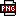此主题相关图片如下：代码执行结果.png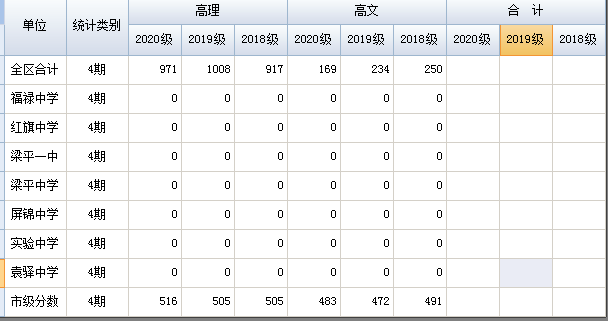[此贴子已经被作者于2019/11/11 11:09:34编辑过]4楼 | 信息 | 搜索 | 邮箱 | 主页 | UC加好友发短信Post By：2019/11/11 11:26:00 [只看该作者]

 看不出什么问题，上传实例说明cqlpjks
5楼 | 信息 | 搜索 | 邮箱 | 主页 | UC加好友发短信Post By：2019/11/11 11:36:00 [只看该作者]

 实例：文件大于2MB，上传不了。6楼 | 信息 | 搜索 | 邮箱 | 主页 | UC加好友发短信Post By：2019/11/11 11:46:00 [只看该作者]

 新建一个项目，导入对应的表以及部分测试数据，然后上传这个新项目即可cqlpjks
7楼 | 信息 | 搜索 | 邮箱 | 主页 | UC加好友发短信Post By：2019/11/11 13:53:00 [只看该作者]

 下载信息  [文件大小：   下载次数： ]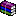点击浏览该文件:管理项目1.rar8楼 | 信息 | 搜索 | 邮箱 | 主页 | UC加好友发短信Post By：2019/11/11 14:24:00 [只看该作者]

 For Each tjdw As String In DataTables("成绩库").GetValues("单位","[年级] = '" & nj & "'And [类别] = '"& tjlb &"'")                dr = DataTables(bm).find("单位='" & tjdw & "'")                If dr Is Nothing Then                    dr = DataTables(bm).addnew()                    dr("单位") = tjdw                End If                dr("统计类别") = tjlb                dr( tjbb & i ) = DataTables("成绩库").Compute("count(单位)", "" & tj1 & " >= '" & wdr("目标" & (i+1) ) & "' And [单位] = '" & dr("单位") & "' And [部别] = '" & tjbb & "' And [类别] = '" & tjlb & "'And [年级] = '"& (nj-i) &"'")            Nextcqlpjks
9楼 | 信息 | 搜索 | 邮箱 | 主页 | UC加好友发短信Post By：2019/11/11 15:22:00 [只看该作者]

 搞定。谢谢！另求：批量设置要显示的列及其列宽：Tables( Value & "重点人数对照表").SetColVisibleWidth("单位|65|统计类别|50|tjbb & i|55|"合计"& i|55")其列名tjbb & i和"合计"& i是变量(tjbb的值是“高文、高理”，i是0 To 2），应如何书写？请指教。谢谢！10楼 | 信息 | 搜索 | 邮箱 | 主页 | UC加好友发短信Post By：2019/11/11 16:02:00 [只看该作者]

 dim str as string = ""for i as integer = 0 to 2str = str & "|" & tjbb & i & "|55|合计" & i & "|55"nextTables( Value & "重点人数对照表").SetColVisibleWidth("单位|65|统计类别|50|" & str.trim("|")) [此贴子已经被作者于2019/11/11 16:02:46编辑过]总数 21 1 2 3 下一页